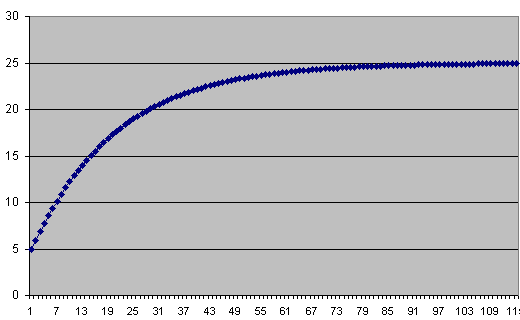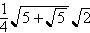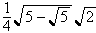Erich Prisner

# Random Walks I

There are 6 adjacent rooms, and a person starting in the first one. Each minute the person goes randomly into an adjacent room. Simulate what happens below.

 Time:

Eventually the person will arrive in the last room, but, as you see, it may take some time. The question is: What is the expected time? In other words: If you perform the experiment very often, say 100 times, and take the average of all resulting times, what would you expect this average to be? Do it with 10 runs to get an impression.

Let' s assume 1000 persons start in the left room and move independently (they don't care how the others move, let's also assume that the rooms are large enough). Then certainly all 1000 will move in the second room, but after one more minute, about 500 will return to the first room, and the others (also about 500) will move on the the third one. And so on. Of course there is still randomness involved, but the large number of people involved will level the randomness (the "law of large numbers"). So what we will do is to analyze the game by assuming that in each room where people have a choice half of them go left and half of them go right. This is done below, where numbers are ratios of people in the room divided by the total number of people. There are two variants: If you click the "step with absorption" button, all people entering the rightmost room are taken out of the simulation---they already "arrived".

Another interpretation is to consider only one person; then the numbers are the probabilities that the person is in this room at the given time.

 Time:Now all we have to do is to click the "step with absorption" button repeatedly and take notes on how many people arrived in the rightmost room in step n. This I did in some Excel sheet you can download. The values of arriving people for the different times are shown in the first graph to the right.

With this incomplete data, the average cannot be computed. What can be computed are mode---the most frequently occuring arrival time, which is both 7 and 9 with frequency 0,078125--- and median---the time when 50% of the persons arrived, which is 19. What can be done is to compute at each step a lower bound for the average. For this we just assume that all people not yet in at that time would arrive in the next step. The various lower bounds, computed at different times, are given in the second graph below.Try random walks on paths of length 4 or length 5. It is fairly easy to see, even without much of the tools used on these pages, that on a path of lebgth 3 the expected value for the transition time from left to right is 4. For the length-4 path, this average transition time turns out to be 9. So is maybe the transition time from left to right in the path of length n equal to (n-1)2? Looking on the data on the Excel sheets also supports this claim.

At least for our length-6 case the conjecture is true. The expected transition time turns out to be 25. Modeling the situation with a system of recurrence relations, we obtain the formula

f(n)=0.06498393925()n- 0.06498393925()n -0.2752763840()n +0.2752763840()n

for the probability to finish in exactly n steps. For even n this equals 0, and for odd n it is

f(n)=0.1299678785 ()n-0.5505527680 ()n.

Therefore the expected transition time equals=

= 0.12997-0.55055=

25.35739 - 0.35739 = 25, using again a series formula.

next page

Erich Prisner 2004